Courses

# Electronic Devices 1

## 10 Questions MCQ Test RRB JE for Electronics & Communication Engineering | Electronic Devices 1

Description
This mock test of Electronic Devices 1 for Electronics and Communication Engineering (ECE) helps you for every Electronics and Communication Engineering (ECE) entrance exam. This contains 10 Multiple Choice Questions for Electronics and Communication Engineering (ECE) Electronic Devices 1 (mcq) to study with solutions a complete question bank. The solved questions answers in this Electronic Devices 1 quiz give you a good mix of easy questions and tough questions. Electronics and Communication Engineering (ECE) students definitely take this Electronic Devices 1 exercise for a better result in the exam. You can find other Electronic Devices 1 extra questions, long questions & short questions for Electronics and Communication Engineering (ECE) on EduRev as well by searching above.
QUESTION: 1

### The Fermi level in a semiconductor bar should

Solution:

Under equilibrium:

- The fermi level across the entire material will be sum and does not vary with distance.

- If there is any disturbance in the material, like junction contact, injection of impurities at any point, the charge carriers redistribute themselves such that the fermi-potential is same in entire material.

Under non-equilibrium:

- The fermi level is uneven with gradient of charge distribution across distance, in material.

- It can be studied using quasi-fermi states, related to charge distribution.

*Answer can only contain numeric values
QUESTION: 2

### Hole mobility in Ge at room temperature is 1900 cm2/V-sec. The diffusion coefficient is ________cm2/sec. (Take kT = 25 mV)

Solution:

Using the relation

D = 0.025 × 1900 cm2/sec

= 47.5 cm2/sec

QUESTION: 3

### In the band diagram of a semiconductor, the fermi level is 0.3 eV above the intrinsic level. The energy level E in the diagram represents.

Solution: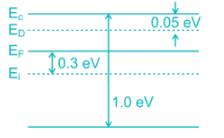Given that fermi level is 0.3 eV above the intrinsic level, hence the type of semiconductor is n-type.

In n-type the donor level is just below the conduction band and above the fermi level.

*Answer can only contain numeric values
QUESTION: 4

A hypothetical semiconductor has bandgap of 0.56 eV at 300 K. If the effective mass of electron in such semiconductor is 4 times that of hole. Then the probability of occupancy of top of valence band (upto 2 decimal) at 300 K is

(Take kT = 26 mV)

Solution:

Position of Fermi level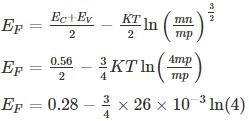EF = 0.28 – 0.027

EF = 0.252 eV

Probability of occupancy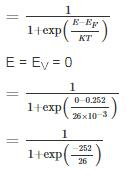≈ 0.99

Note:

This signifies that at room temperature the top of valence band is almost filled

QUESTION: 5

The Difference between the donor energy level and fermi level in a n-type semiconductor in where 25% of the atoms are ionised at 300 k is

Solution:

As 25% of donor atoms are ionised, the occupation probability of donor level is 0.75.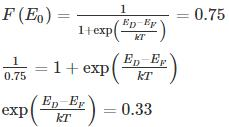ED - EF = -0.028 eV

*Answer can only contain numeric values
QUESTION: 6

A semiconductor with intrinsic carrier concentration 1 × 1010 cm-3 at 300°K has both valence and conduction band effective densities of states NC and NV equal to 1019 cm-3. The band gap Eg is _____ eV.

Solution:

The intrinsic concentration is related to bandgap by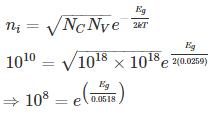Eg = 0.0518 ln (108) eV

= 0.954 eV

*Answer can only contain numeric values
QUESTION: 7

In a very long p-type Si bar with doping concentration Na = 1017 cm-3, excess holes are injected such that excessive concentration of holes at x = 0 is 5 × 1016 cm-3.

The hole concentration at x = 1 μm is _____ × 1017 cm-3. Take μp = 500 cm2/v-s and recombination time constant τ = 10-8 s, kT = 0.0259 eV

Solution:

Diffusion constant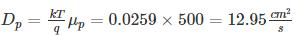⇒ Diffusion length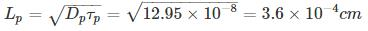Hole concentration at any distance x is sum of equillibrium and excess hole concentration

excess hole concentration varies with the distance x as Δp e-x/Lp

p = p + Δp e-x/Lp

Where po = Doping concentration

Δp = extra hole concentration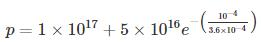= (1+.379) x 1017

1.379 × 1017 cm-3

QUESTION: 8

In an intrinsic semiconductor, the Fermi energy level EF doesn’t lie in the middle of the band gap cause

Solution:

In an intrinsic semi-conductor the Fermi energy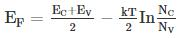Where NC and NV are density of state in valence and conduction bond respectively.

Since NC ∝ mn, effective mass of electron and Nv ∝ mp, effective mass of hole
Since mn and mp are not equal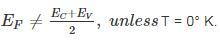QUESTION: 9

A silicon bar is doped with donor impurities ND = 2.25 × 1015 cm-3

If the electron mobility μn = 1000 cm2/v-s then the approximate value of resistivity of silicon bar assuming partial ionization of 55% is __________ (Ω - cm)

Solution:

Given ND = 2.25 × 1015 cm-3

Partial ionization of 55%, number of electrons is

⇒ n = 0.55 × 2.25 × 1015 cm-3

= 1.2375 × 1015 cm-3

Electron mobility μn = 1000 cm2/v-s

Resistivity of n-type silicon =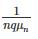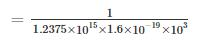= 5.05 Ω – cm

QUESTION: 10

Holes are injected into n-type Ge so that the at the surface of the semiconductor hole concentration is 1014/cm3. If diffusion constant of a hole in Ge is 49cm2/sec and minority carrier lifetime is τp = 10-3 sec. Then the hole concentration Δp at a distance of 4mm from the surface is ______1014/cm3

Solution: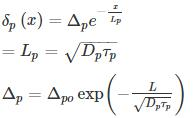= 1.6 × 1013/cm3

= 0.16 × 1014/cm3